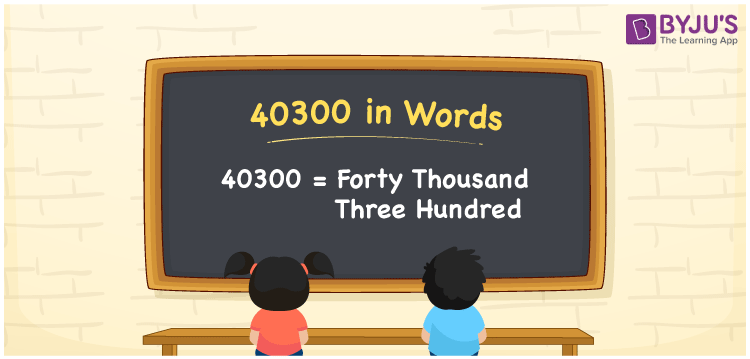# 40300 in Words

40300 in words is written as Forty thousand three hundred. In both the International System of Numerals and the Indian System of Numerals, 40300 in words is written as Forty thousand three hundred. The number 40300 is a Cardinal Number as it represents some quantity. For example, “she has 40300 rupees in her bank account”.

 40300 in Words Forty thousand three hundred Forty thousand three hundred in Number 40300

## 40300 in English Words

40300 in English words is read as “Forty thousand three hundred”.## How to Write 40300 in Words?

To write 40300 in words, we shall use the place value chart. In the place value chart, put 4 in the ten thousands, 0 in the thousands, 3 in the hundreds, 0 in the tens and ones, respectively. Let us make a place value chart to write the number 40300 in words.

 Ten Thousands Thousands Hundreds Tens Ones 4 0 3 0 0

Thus, we can write the expanded form as

4 × Ten Thousand + 0 × Thousand + 3 × Hundred + 0 × Ten + 0 × One

= 4 × 10000 + 0 × 1000 + 3 × 100 + 0 × 10 + 0 × 1

= 40000 + 0 + 300 + 0 + 0

= 40300

= Forty thousand three hundred.

40300 is a natural number that lies between 40299 and 40301.

40300 in words – Forty thousand three hundred

• Is 40300 an odd number? – No
• Is 40300 an even number? – Yes
• Is 40300 a perfect square number? – No
• Is 40300 a perfect cube number? – No
• Is 40300 a prime number? – No
• Is 40300 a composite number? – Yes

## Frequently Asked Questions on 40300 in Words

Q1

### How to write 40300 in words?

40300 in words is written as Forty thousand three hundred.
Q2

### How to write 40300 in the International and Indian System of Numerals?

In both, the system of numerals, 40300 in words, is written as Forty thousand three hundred.
Q3

### How to write 40300 in a place value chart?

In the place value chart, write 4 in the ten thousands, 0 in the thousands, 3 in the hundreds, 0 in the tens and ones, respectively.